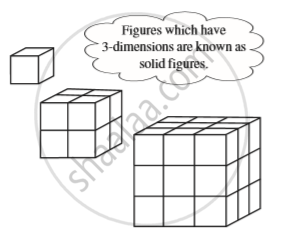Share

# Introduction of Cubes and Cube Root

Course

#### notes

The word ‘cube’ is used in geometry. A cube is a solid figure which has all its sides equal.Consider the numbers 1, 8, 27, ...
These are called perfect cubes or cube numbers.
We note that 1 = 1 × 1 × 1 = 1^3;  8 = 2 × 2 × 2 = 2^3;
27 = 3 × 3 × 3 = 3^3.
The following are the cubes of numbers from 1 to 10.

 Number Cube 1 1^3 = 1 2 2^3 = 8 3 3^3 = 27 4 4^3 = 64 5 5^3 = 125 6 6^3 = 216 7 7^3 = 343 8 8^3 = 512 9 9^3 =729  10 10^3 = 1000

### Shaalaa.com

Cubes and Cube Roots [00:11:31]
S
0%

S